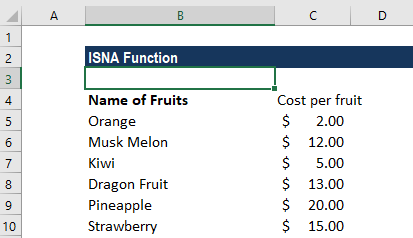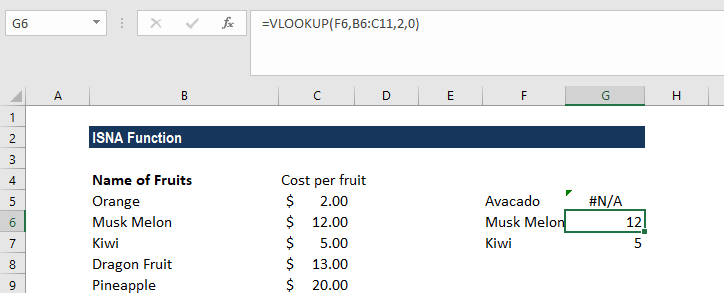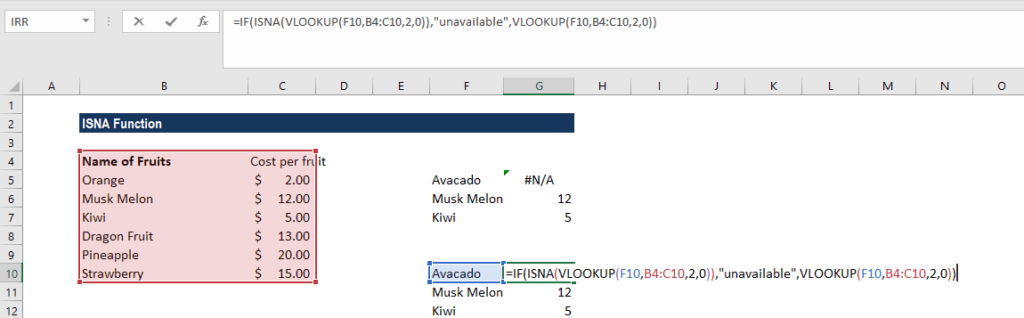# ISNA Function

Checks if a cell contains the #N/A! error

## What is the ISNA Excel Function?

The ISNA Excel function is categorized under Information functions. This function helps in checking if a cell contains the #N/A! error. It will return a FALSE value for other types of errors.

As a financial analyst, we deal with data and perform analysis on it. The ISNA function helps to identify cells that contain an #N/A error and facilitates smooth comparisons, data analysis, etc.

We can use the ISNA function with the IF function and test a cell for error and display a customized message for it.

### Formula

=ISNA(value)

The ISNA Excel function uses the following argument:

1. Value (required argument) – This is the expression or value that needs to be tested. It is generally provided as a cell address.

The ISNA function will return TRUE if the value is an #N/A error and FALSE if it is not.

### How to use the ISNA Function in Excel?

To understand the uses of the ISNA function, let’s consider a few examples:

#### Example 1

Let’s first understand how the ISNA Excel function behaves using the following set of data:

DataFormulaResultRemarks
#DIV/0!=ISNA(#DIV0!)FALSEAlthough the expression given was an error, it is not the #N/A error. The ISNA function returned FALSE.
#N/A=ISNA(#N/A)TRUEThe ISNA function returned TRUE, as it is an #N/A error.
#NAME?=ISNA(#NAME?)FALSEAlthough the expression given was an error, it is not the #N/A error. The ISNA function returned FALSE.
Text=ISNA(TEXT)FALSEThe ISNA function doesn’t cover text so it returned FALSE.

#### Example 2

Suppose we wish to find out the cost of a few fruits. When using the VLOOKUP formula, one of the irritating things is seeing the “#N/A” error after Excel determined a lookup value is not available.

Rather than showing values when they are unavailable, from a visual design perspective, it’s better to just show a blank space or a “Not found” message. It makes our output look more professional and polished. It also draws less attention to the error values and lets users focus on the values found. So, we want MS Excel to return a customized message, “Not found”, when it is unable to find a fruit from the given list instead of #N/A!.

Suppose we are given the data below:When we did a VLOOKUP for the given list of fruits, we got the list below:Now instead of the #N/A error, we want a customized message that says “Unavailable.”

The formula to use will be:We need to drag the formula for the entire list for which we need to find out the cost. Using the IF-ISNA formula combination is easy. All we need to do is to wrap the ISNA formula inside an IF logic condition. Remember, the crucial thing here while using the IF-ISNA formula combination is that we need to put in the original formula twice.

We will get the result below:

### Things to remember about the ISNA Excel Function

1. The ISNA function is part of the IS functions that are often used to test the results of formulas in a cell for errors.
2. If we are using MS Excel 2013-2016, the function is very useful for identifying and handling #N/A! errors.

Thanks for reading CFI’s guide to important Excel functions! By taking the time to learn and master these functions, you’ll significantly speed up your financial analysis. To learn more, check out these additional CFI resources:

• Excel Functions for Finance